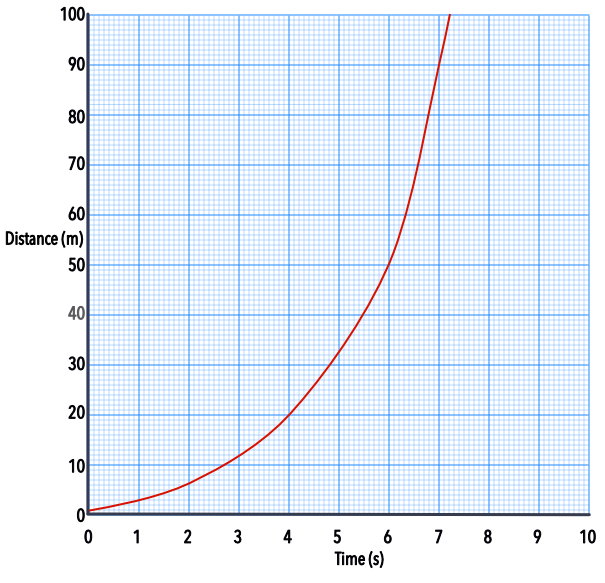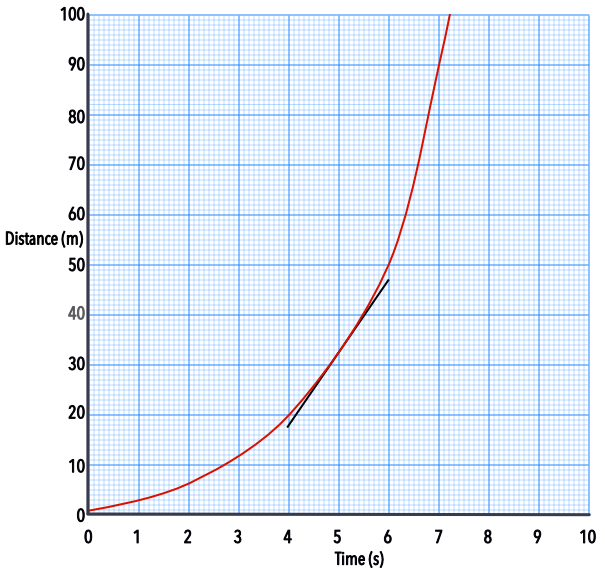Rates of Change in Context

# Rates of Change in Context

GCSE(H),

Rates of Change may be determined graphically, using a chord or tangent to a graph; or numerically, by using known numbers. Other uses for determining rates of change include calculating flow of water through pipes, train scheduling, fuel consumption in engines and factory production.

## Examples

1. A racing car accelerates from stationary and covers 260m in the first six seconds. In the following six seconds, it covers a further 460m. What is the average velocity of the car over the first 12 seconds?

Total distance covered is 260 + 460 = 720m

Total time = 12 seconds

Average velocity = frac(text(distance))(text(time)) = 720 ÷ 12 = 60m/s

2.The graph shows distance against time for a new locomotive. What is the speed of the locomotive at 5 seconds?gradient = frac(text(up))(text(along)) = frac(47 - 18)(6 - 4) = frac(29)(2) = 14.5 m/s